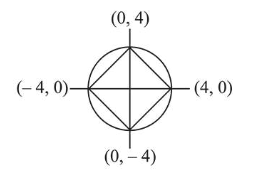# If z be a complex number satisfyingQuestion:

If $z$ be a complex number satisfying $|\operatorname{Re}(z)|+|\operatorname{Im}(z)|=4$, then $|z|$ cannot be:

1. (1) $\sqrt{\frac{17}{2}}$

2. (2) $\sqrt{10}$

3. (3) $\sqrt{7}$

4. (4) $\sqrt{8}$

Correct Option: , 3

Solution:

$z=x+i y$

$|x|+|y|=4$

$|z|=\sqrt{x^{2}+y^{2}}$Minimum value of

$|z|=4$

$|z| \in[\sqrt{8}, \sqrt{16}]$

So, $|z|$ can't be $\sqrt{7}$